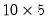Command Reference : Matrix Language : Matrix Views and Procs

Matrix Views and Procs
The individual object descriptions list the various views and procs for the various matrix objects. Listings are available for matrices ( “Matrix”), vectors ( “Vector”), symmetric matrices ( “Sym”), rowvectors ( “Rowvector”), and coefs ( “Coef”).
Matrix Graph and Statistics Views
All of the matrix objects, with the exception of the scalar object, have windows and views. For example, you may display line and bar graphs for each column of thematrix Z:
z.line
z.bar(p)
Each column will be plotted against the row number of the matrix.
Additionally, you can compute descriptive statistics for each column of a matrix, as well as the correlation and covariance matrix between the columns of the matrix:
z.stats
z.cor
z.cov
By default, EViews performs listwise deletion by column when computing correlations and covariances, so that each group of column statistics is computed using the largest possible set of observations.
The full syntax for the commands to display and print these and other views is provided in the reference for the specific object (e.g., matrix, sym) Object Reference.
Matrix Input and Output
EViews provides you with the ability to read and write files directly from matrix objects using the import and export procedures.
Import
You may read directly into an EViews matrix object (matrix, vector, sym, etc.) from text (both ASCII and binary), HTML, Excel XLSX, and Excel 97 XLS files. Excel reads includes support for named ranges and multiple pages. The new engine supports a number of different formats.
Excel Example
The command
matrix_name.import "c:\data files\data.xls"
loads the active sheet of DATA.XLSX into the MATRIX_NAME matrix object.
matrix_name.import "c:\data files\data.xls" range="GDP data"
reads the data contained in the “GDP data” sheet of “Data.XLS” into the MATRIX_NAME object.
HTML Example
The command
mat1.import "c:\data.html"
loads into the MAT1 matrix object the data located in the HTML file “Data.HTML” located on the C:\ drive
loads into a matrix object MAT1 the data with the given URL located on the website site “http://www.tradingroom.com.au”. The column header is set to three rows.
Text Example
The command
mat2.import c:\data.csv skip=5
reads “Data.CSV” into a MAT2, skipping the first 5 rows.
mat2.import(type=text) c:\date.txt delim=comma
loads the comma delimited data “Date.TXT” into the MAT2 matrix object.
Export
The export command supports a number of formats including the various ASCII, binary, HTML, RTF, and Excel formats, along with LaTeX, Markdown, and PDF files. Importantly, the Excel XLSX export allows you to write the matrix results into existing Excel files, beginning at a specified cell.
The command:
matrix1.export mymatrix
exports the data in MATRIX1 to a CSV file named “mymatrix.CSV” in the default directory.
matrix1.export(h, t=csv, n="NaN") mymatrix
saves the contents of MATRIX1 along with the column and row headers to a CSV (comma separated value) file named “mymatrix.CSV” and writes all NA values as “NaN”.
matrix1.export(h, t=html, s=50) mymatrix
exports the data in MATRIX1 along with the column and row headers to a HTML file named “mymatrix.HTM” at half of the original size.
matrix1.exports(n=".", r=B) mymatrix
saves the data in the second column to a CSV file named “mymatrix.CSV”, and writes all NA values as “.”.
matrix1.export(t=excelxml, cellfmt=clear, mode=update) mymatrix range=Country!b5
writes the data in MATRIX1 to the preexisting “mymatrix.XLSX” Excel file to the “Country” sheet at cell B5, where all cell formatting is cleared.
There are many more options for controlling reading and writing of matrix data; “Basic Data Handling” offers extensive discussion. See also the descriptions for the matrix procs Matrix::import and Matrix::export